##### The Japanese Bonsai specialist
Direct order Contact Help / Services Newsletter# Hosta sunshine glory

Hosta sunshine glory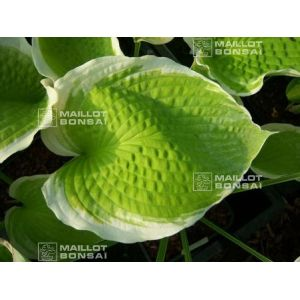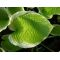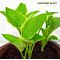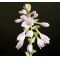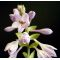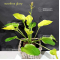ref. : 7142

6,00

Available quantity : 8Order

###### Description

'Sunshine Glory' is an outstanding hosta that becomes a nearly upright mound of foliage with wide, creamy white margins. It makes an ideal plant for the middle or back of a shaded border. The leaf centre changes from gold to pale green during the growing season and the foliage is moderately corrugated and wavy. Lavender flowers bloom in June and July.

Height: 50 cm x 100 cm wide.
Leaves 25 cm long x 15 cm wide.

Derived from crossing a 'William Lachman' with 'Golden Waffles'.

Resistant to slugs and snails' attacks.

Big rhizome delivered in a 1.5 litre plastic container.

#sunshine 3.8 #hosta 3.5 #glory 3.5 #wide 3.4 #foliage 2.7 #hostas 2.6 #with 2.4 #from 2.4 #outstanding 2.1 #moderately 2

###### Technical description
 height 50-70 cm

Formule
(( ROUND((CHAR_LENGTH(b.article_nom)-CHAR_LENGTH(REPLACE(b.article_nom, 'sunshine', '')))/LENGTH('sunshine')) + ROUND((CHAR_LENGTH(b.article_description)-CHAR_LENGTH(REPLACE(b.article_description, 'sunshine', '')))/LENGTH('sunshine')) ) * 3.8) + (( ROUND((CHAR_LENGTH(b.article_nom)-CHAR_LENGTH(REPLACE(b.article_nom, 'hosta', '')))/LENGTH('hosta')) + ROUND((CHAR_LENGTH(b.article_description)-CHAR_LENGTH(REPLACE(b.article_description, 'hosta', '')))/LENGTH('hosta')) ) * 3.5) + (( ROUND((CHAR_LENGTH(b.article_nom)-CHAR_LENGTH(REPLACE(b.article_nom, 'glory', '')))/LENGTH('glory')) + ROUND((CHAR_LENGTH(b.article_description)-CHAR_LENGTH(REPLACE(b.article_description, 'glory', '')))/LENGTH('glory')) ) * 3.5) + (( ROUND((CHAR_LENGTH(b.article_nom)-CHAR_LENGTH(REPLACE(b.article_nom, 'wide', '')))/LENGTH('wide')) + ROUND((CHAR_LENGTH(b.article_description)-CHAR_LENGTH(REPLACE(b.article_description, 'wide', '')))/LENGTH('wide')) ) * 3.4) + (( ROUND((CHAR_LENGTH(b.article_nom)-CHAR_LENGTH(REPLACE(b.article_nom, 'foliage', '')))/LENGTH('foliage')) + ROUND((CHAR_LENGTH(b.article_description)-CHAR_LENGTH(REPLACE(b.article_description, 'foliage', '')))/LENGTH('foliage')) ) * 2.7) + (( ROUND((CHAR_LENGTH(b.article_nom)-CHAR_LENGTH(REPLACE(b.article_nom, 'with', '')))/LENGTH('with')) + ROUND((CHAR_LENGTH(b.article_description)-CHAR_LENGTH(REPLACE(b.article_description, 'with', '')))/LENGTH('with')) ) * 2.4) + (( ROUND((CHAR_LENGTH(b.article_nom)-CHAR_LENGTH(REPLACE(b.article_nom, 'from', '')))/LENGTH('from')) + ROUND((CHAR_LENGTH(b.article_description)-CHAR_LENGTH(REPLACE(b.article_description, 'from', '')))/LENGTH('from')) ) * 2.4) + (( ROUND((CHAR_LENGTH(b.article_nom)-CHAR_LENGTH(REPLACE(b.article_nom, 'outstanding', '')))/LENGTH('outstanding')) + ROUND((CHAR_LENGTH(b.article_description)-CHAR_LENGTH(REPLACE(b.article_description, 'outstanding', '')))/LENGTH('outstanding')) ) * 2.1) + (( ROUND((CHAR_LENGTH(b.article_nom)-CHAR_LENGTH(REPLACE(b.article_nom, 'moderately', '')))/LENGTH('moderately')) + ROUND((CHAR_LENGTH(b.article_description)-CHAR_LENGTH(REPLACE(b.article_description, 'moderately', '')))/LENGTH('moderately')) ) * 2) + (( ROUND((CHAR_LENGTH(b.article_nom)-CHAR_LENGTH(REPLACE(b.article_nom, 'corrugated', '')))/LENGTH('corrugated')) + ROUND((CHAR_LENGTH(b.article_description)-CHAR_LENGTH(REPLACE(b.article_description, 'corrugated', '')))/LENGTH('corrugated')) ) * 2)

## Secure payment## Delivery

Our logistic partners :04 74 55 23 48
Pépinière MAILLOT-BONSAÏ
Le Bois Frazy
01990 RELEVANT - FRANCE
on appointment